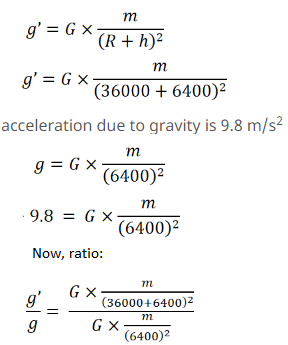# HC Verma Solutions Class 11 Chapter 4 The Forces

HC Verma Solutions for class 11 chapter 4 – the forces are available for physics students who may be having trouble in understanding and solving problems related to the chapter. By preparing for physics topics like these, students are also simultaneously preparing themselves for the IIT JEE exams as well. The HC Verma Solutions vol 1 for chapter 4 explains the topics about the different forces that are occurring in our world around us. Important topics such as the force of gravitation, the force of attraction are some of the important forces that govern the laws of physics here on planet Earth. With the help of these solutions, students learn about the different forces that govern our universe. Students can also increase their problem-solving efficiency by referring to the solved examples and practising them. This chapter also teaches students about interesting concepts which are utilised in the numerical problems such as Mass of Earth, Weight of Earth, Mass of Satellite, Radius of Earth, Coulomb’s Force etc.

Some of the main topics discussed in this chapter are:

1. Gravitational Force
2. Electromagnetic (EM) Force
3. Nuclear Forces
4. Weak Forces
5. Scope of Classical Physics

## Class 11 Important Question In Chapter 4

1. You are holding a pen and writing in a notebook. Do you exert any force on the pen? Explain the kind of force. Also, elucidate the force exerted by the pen on the notebook and the force exerted by you on the notebook?
2. During a meetup, Neils Bohr and Werner Heisenberg shook hands with each. What is the type of force they exerted?
(a) Weak (b) Nuclear (c) Electromagnetic (d) Gravitational.
3. Classical physics adequately describes which situation perfectly?
(a) a hydrogen atom.
(b) the motion of a dust particle.
(c) a neutron changing to a proton.
(d) the motion of a cricket ball.
4. Do you agree when it is said that the reaction of a gravitational force is always gravitational or an electromagnetic force is always electromagnetic?
5. A man who is 60 kg carries and moves a man weighing 40 kg man using a force of 60 N.
What force has the 40 kg man used to push the other man?
(a) 0 (b) 40 N (c) 20 N (d) 60 N.

## HC Verma Solutions Vol 1 The Forces Chapter 4

Question 1: The gravitational force acting on a particle of 1g due to a similar particle is equal to 6.67 × 10–17 N. Calculate the separation between the particles.

Solution:

Mass of the particle, m = 1 g or 1/1000 kg

Let the r be the distance between the particles, then the gravitational force between the particles,

F = 6.67 x 10-17 N

Now, gravitational force existing between the particles: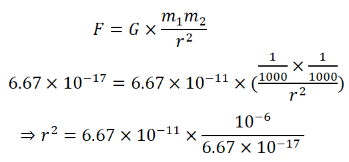=> r = 1m

Separation between the particles is 1 m.

Question 2: Calculate the force with which you attract the earth.

Solution:

Let m be the mass of the man standing on earth, the earth’s gravitational force will act on man mg.

Where m = mass of the man and g = acceleration due to gravity on the surface of earth =10 m/s2

Let mass of the man=50 kg.

F = W = mg = 50×10 = 500N (force acting on the man)

Man is also attracting the earth with a force of 500 N.

Question 3: At what distance should two charges, each equal to 1 C, be placed so that the force between them equals your weight?

Solution:

Coulomb’s force of attraction between two charges is given by –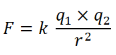Where, q1 and q2 are the charges, r= distance between the charges and k = proportionality constant

Here q1 = q2 = 1C and k = 9 × 109

=> F = k/r2 …(1)

Force of attraction is equal to the weight (Given)

F = mg …(2)

Equation (1) and (2), we have

mg = k/r2 …(3)

Let us assume that, mass = m = 64 kg

(3)=> r = (3×104)/√64

r = 3750 m

Question 4: Two spherical bodies, each of mass 50 kg, are placed at a separation of 20 cm. Equal charges are placed on the bodies and it is found that the force of Coulomb repulsion equals the gravitational attraction in magnitude. Find the magnitude of the charge placed on either body.

Solution:

Given, mass = 50 kg, r = 20 cm or 0.2 m

Coulomb’s force of repulsion between two charges: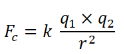Where, q1 and q2 are the charges, r= distance between the charges and k = proportionality constant

Here q1 = q2 = 1C and k = 9 × 109

Again, gravitational force,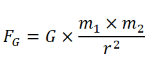Where, G = universal gravitation constant

m1 = mass of first object and m2 = mass of second object and r = distance between the objects

m1 = m2 = 50Kg

Now,

FG = FC

=>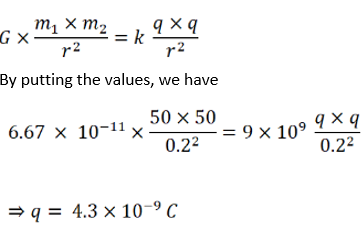Question 5: A monkey is sitting on a tree limb. The limb exerts a normal force of 48 N and a frictional force of 20 N. Find the magnitude of the total force exerted by the limb on the monkey.

Solution:

Given: Normal force = 48N and Frictional force = 20N

The resultant magnitude of the force, say R,

R2 = (48) 2 + (20) 2

or R = 52 N

Question 6: A body builder exerts a force of 150 N against a bullworker and compresses it by 20 cm. Calculate the spring constant of the spring in the bullworker.

Solution:

Body builder exerts a force = 150N

Compressed length = 20cm or 0.2m

Force applied on a string: F = kx

Where, K = spring constant and x = displacement

150 = k x 0.2

=> k = 750 N/m

Question 7: A satellite is projected vertically upwards from an earth station. At what height above the earth’s surface will the force on the satellite due to the earth be reduced to half its value at the earth station? (Radius of the earth is 6400 km)

Solution:

Given, radius of Earth = 6400 Km

Let R be the radius of earth, M be the mass of earth and m be the mass of the satellite.

Also assume, at height h, the force on the satellite due to earth is reduce to half.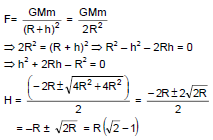= 6400 x 0.414

= 2650 km

Question 8: Two charged particles placed at a separation of 20 cm exert 20 N of Coulomb force on each other. What will be the force if the separation is increased to 25 cm?

Solution:

Coulomb’s force between two charges: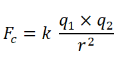Where, q1 and q2 are the charges, r= distance between the charges and k = proportionality constant

and k = 9 × 109

When, r = 20 cm = 0.2m, Coulomb’s force = 20 N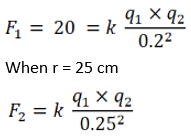Now, ratio of F1 and F2

F1/F2 = 20/F2 = (0.2)2/(0.25) 2

=> F2 = 13 N (approx)

Question 9: The force with which the earth attracts an object is called the weight of the object. Calculate the weight of the moon from the following data: The universal constant of gravitation G = 6.67 × 10–11 N–m2/kg2, mass of the moon = 7.36 × 1022 kg, mass of the earth = 6 × 1024 kg and the distance between the earth and the moon = 3.8 × 105 km.

Solution:

mass of the moon = 7.36 × 1022 kg

mass of the earth = 6 × 1024 kg

distance between moon and earth =3.8 × 105 km

The force between moon and earth: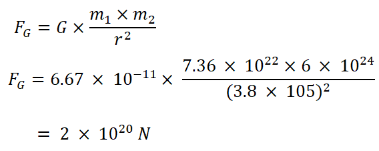Weight of the moon is 2.0 × 1020 N.

Question 10: Find the ratio of the magnitude of the electric force to the gravitational force acting between two protons.

Solution:

Coulomb’s force of repulsion between two protons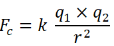and gravitational force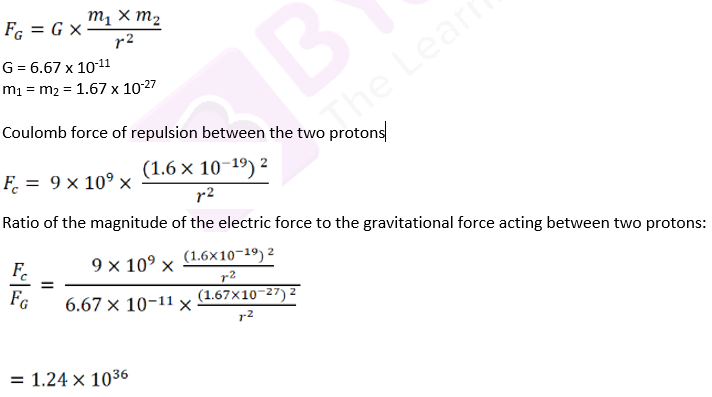Question 11: The average separation between the proton and the electron in a hydrogen atom in ground state is 5.3 × 10–11 m. (a) Calculate the Coulomb force between them at this separation. (b) When the atom goes into its first excited state the average separation between the proton and the electron increases to four times its value in the ground state. What is the Coulomb force in this state?

Solution:

The average separation between the proton and the electron of a Hydrogen atom in ground state, r = 5.3 × 10−11 m

(a) Coulomb’s force of between electron and protonWhere, q1 and q2 are the charges, r= distance between the charges and k = proportionality constant

and k = 9 × 109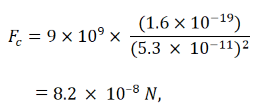(b) the Coulomb force when the average separation between the proton and the electron increases to four times its value in the ground state: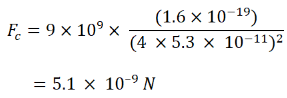Question 12: The geostationary orbit of the earth is at a distance of about 36000 km from the earth’s surface. Find the weight of a 120-kg equipment placed in a geostationary satellite. The radius of the earth is 6400 km.

Solution:

The radius of the earth = 6400 km.

Equipment weight = 120-kg

Also given, geostationary orbit of the earth is at a distance of about 36000 km from the earth’s surface.

The value acceleration due to gravity above the surface of the Earth